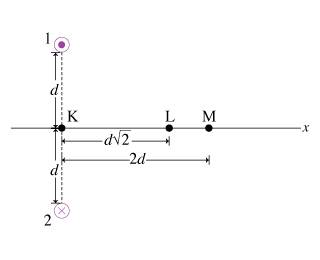# Magnetic field from two wires and magnitude

## Homework Statement

Each of the wires carries a current of magnitude I. The current in wire 1 is directed out of the page and that in wire 2 is directed into the page. The distance between the wires is 2d. The x axis is perpendicular to the line connecting the wires and is equidistant from the wires.

a.) Point M is located a distance 2d from the midpoint between the two wires. Find the magnitude of the magnetic field B_1M created at point M by wire 1.

b.)Find the magnitude of the net magnetic field B_M created at point M by both wires.

## Homework Equations## The Attempt at a Solution

I got the B1L created by wire 1 by point 1 only.
so I thought since they have the same current and distance, they will have the same magnitude, so I just add them. But I got it wrong.
one of the hint says "find the direction of B"
I am confused how to find the direction for wire 1 and 2 at point M.